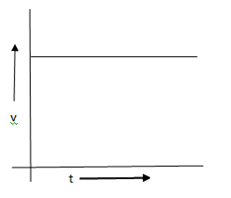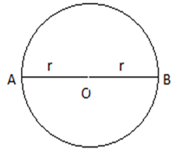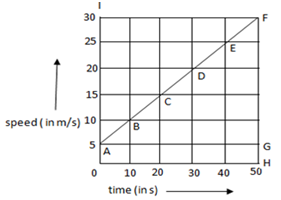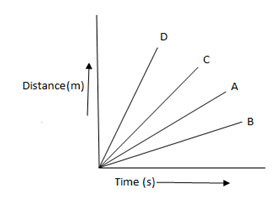# MCQ Questions for Class 9 Science Chapter 8 Motion with Answers

## MCQ Questions for Class 9 Science Chapter 8 Motion with Answers

MCQs from Class 9 Science Chapter 8 – Motion are provided here to help students prepare for their upcoming Science exam.

MCQs from CBSE Class 9 Science Chapter 8: Motion

1. If the displacement of an object is proportional to square of time, then the object moves with:
(a) Uniform velocity
(b) Uniform acceleration
(c) Increasing acceleration
(d) Decreasing acceleration

(b) Uniform acceleration

2. From the given v-t graph, it can be inferred that the object is(a) At rest
(b) In uniform motion
(c) Moving with uniform acceleration
(d) In non-uniform motion

(b) In uniform motion

3. Suppose a boy is enjoying a ride on a marry-go-round which is moving with a constant speed of 10 m/s. It implies that the boy is:
(a) At rest
(b) Moving with no acceleration
(c) In accelerated motion
(d) Moving with uniform velocity

(c) In accelerated motion

4. A particle is moving in a circular path of radius r.The displacement  after half a circle would be:
(a) Zero
(b) πr
(c) 2r
(d) 2πr

(c) 2r

5. Which of the following can sometimes be ‘zero’ for a moving body?
i. Average velocity
ii. Distance travelled
iii. Average speed
iv. Displacement
(a) Only (i)
(b) (i) and (ii)
(c) (i) and (iv)
(d) Only (iv)

(c) (i) and (iv)

6. Which of the following statement is correct regarding velocity and speed of a moving body?
(a) Velocity of a moving body is always higher than its speed
(b) Speed of a moving body is always higher than its velocity
(c) Speed of a moving body is its velocity in a given direction
(d) Velocity of a moving body is its speed in a given direction

(d) Velocity of a moving body is its speed in a given direction

7. When a car driver travelling at a speed of 10 m/s applies brakes and brings the car to rest in 20 s, then the retardation will be:
(a) + 2 m/s2
(b)  − 2 m/s2
(c)  − 0.5 m/s2
(d) + 0.5 m/s2

(d) + 0.5 m/s2

8. The speed – time graph of a car is given here. Using the data in the graph calculate the total distance covered by the car.(a) 1250 m
(b) 875 m
(c) 1500 m
(d) 870 m

(b) 875 m

9. A car of mass 1000 kg is moving with a velocity of 10 m/s. If the velocity-time graph for this car is a horizontal line parallel to the time axis, then the velocity of the car at the end of 25 s will be:
(a) 40 m/s
(b) 25 m/s
(c) 10 m/s
(d) 250 m/s

(c) 10 m/s

10. Which of the following is most likely not a case of uniform circular motion?
(a) Motion of the earth around the sun
(b) Motion of a toy train on a circular track
(c) Motion of a racing car on a circular track
(d) Motion of hours’ hand on the dial of a clock

(c) Motion of a racing car on a circular track

11. In which of the following cases of motions, the distance moved and the magnitude of the displacement are equal?
i. If the car is moving on a straight road
ii. If the car is moving in circular path
iii. The pendulum is moving to and fro
iv. The earth is moving around the sun
(a) only(ii)
(b) (i) and (iii)
(c) (ii) and (iv)
(d) only (i)

(d) only (i)

12. A car is travelling at a speed of 90 km/h. Brakes are applied so as to produce a uniform acceleration of – 0.5 m/s2. Find how far the car will go before it is brought to rest?
(a) 8100 m
(b) 900 m
(c) 625 m
(d) 620 m

(c) 625 m

13. In a free fall the velocity of a stone is increasing equally ion equal intervals of time under the effect of gravitational force of the earth. Then what can you say about the motion of this stone? Whether the stone is having:
(a) Uniform acceleration
(b) Non-uniform acceleration
(c) Retardation
(d) Constant speed

(a) Uniform acceleration

14. The numerical ratio of displacement to distance for a moving object is:
(a) Always less than 1
(b) Equal to 1 or less than 1
(c) Always more than 1
(d) Equal to 1 or more than one

(b) Equal to 1 or less than 1

15. Four cars A, B, C and D are moving on a levelled, straight road. Their distance time graphs are shown in the figure below. Which of the following is the correct statement regarding the motion of these cars?(a) Car A is faster than car D
(b) Car B is the slowest
(c) Car D is faster than car C
(d) Car C is the slowest